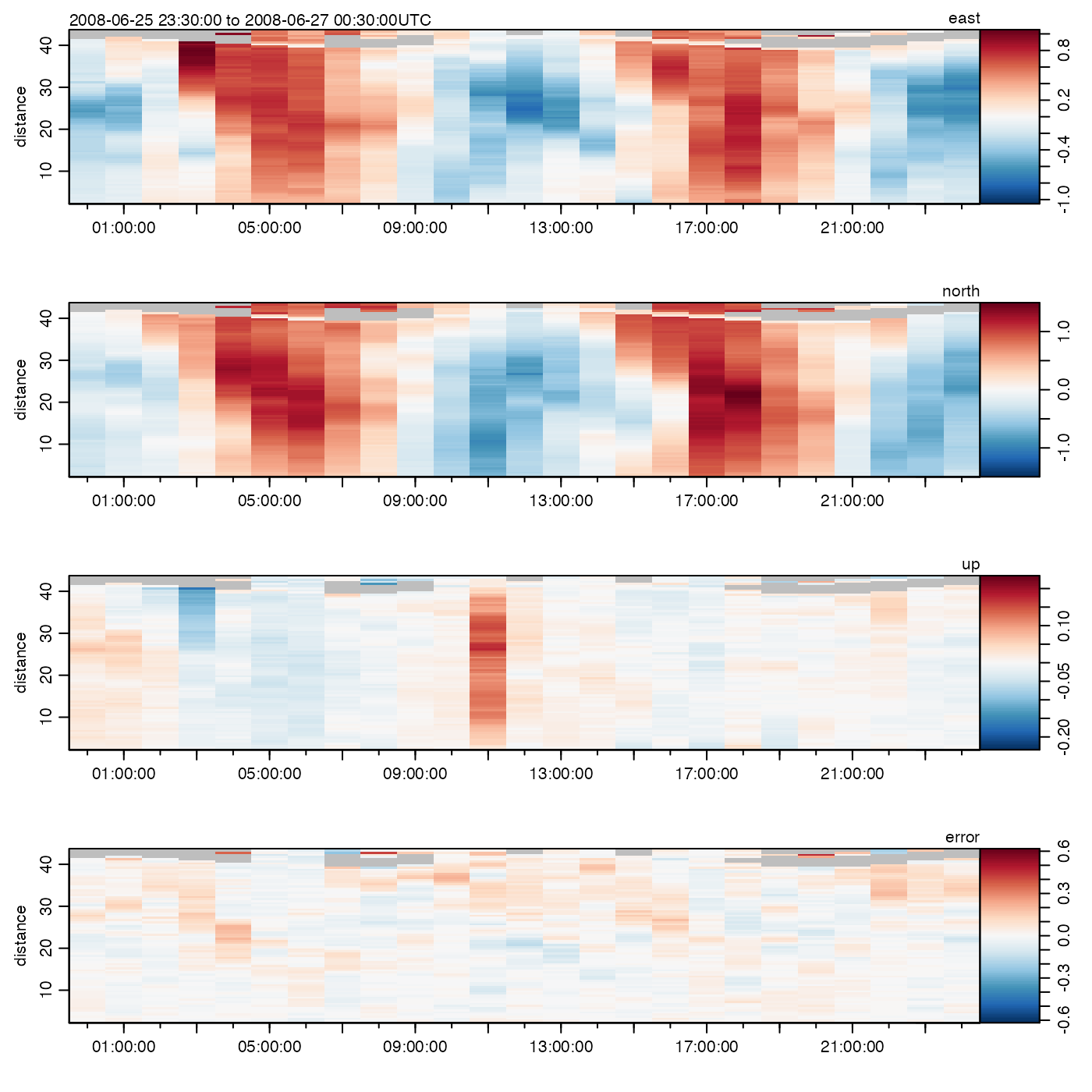Later on, other methods will be added, and ctdDecimate() will be retired in favour of this, a more general, function. The filtering is done with the filter() function of the stats package.

decimate(x, by = 10, to, filter, debug = getOption("oceDebug"))

## Arguments

x

an oce object.

by

an indication of the subsampling. If this is a single number, then it indicates the spacing between elements of x that are selected. If it is two numbers (a condition only applicable if x is an echosounder object, at present), then the first number indicates the time spacing and the second indicates the depth spacing.

to

Indices at which to subsample. If given, this over-rides by.

filter

optional list of numbers representing a digital filter to be applied to each variable in the data slot of x, before decimation is done. If not supplied, then the decimation is done strictly by sub-sampling.

debug

a flag that turns on debugging. Set to 1 to get a moderate amount of debugging information, or to 2 to get more.

## Value

An oce object that has been subsampled appropriately.

## Bugs

Only a preliminary version of this function is provided in the present package. It only works for objects of class echosounder, for which the decimation is done after applying a running median filter and then a boxcar filter, each of length equal to the corresponding component of by.

Filter coefficients may be calculated using makeFilter(). (Note that ctdDecimate() will be retired when the present function gains equivalent functionality.)

Dan Kelley

## Examples

library(oce)